Home » What is Bootstrap Sampling in Statistics and Machine Learning?

# What is Bootstrap Sampling in Statistics and Machine Learning?

## Introduction

Have you ever struggled to improve your rank in a machine learning hackathon on DataHack or Kaggle? You’ve tried all your favorite hacks and techniques but your score refuses to budge. I’ve been there and it’s quite a frustrating experience!

This is especially relevant during your initial days in this field. We tend to go with the familiar techniques that we’ve learned, like linear regression, logistic regression, and so on (depending on the problem statement).

And then along comes Bootstrap Sampling. It is a powerful concept that propelled my rank towards the upper echelons of these hackathon leaderboards. And it was quite a learning experience!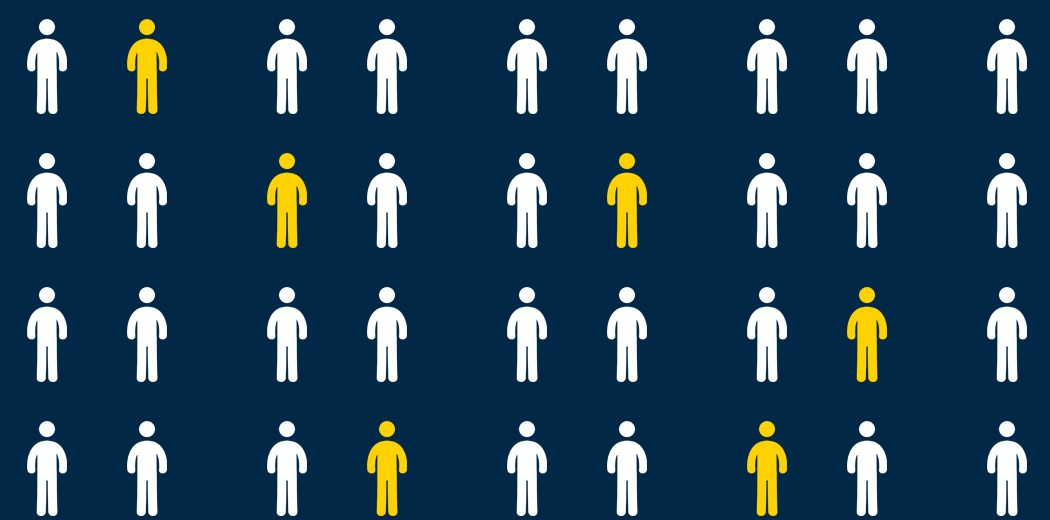Bootstrap sampling is a technique I feel every data scientist, aspiring or established, needs to learn.

So in this article, we will learn everything you need to know about bootstrap sampling. What it is, why it’s required, how it works, and where it fits into the machine learning picture. We will also implement bootstrap sampling in Python.

## What is Bootstrap Sampling?

Here’s a formal definition of Bootstrap Sampling:

In statistics, Bootstrap Sampling is a method that involves drawing of sample data repeatedly with replacement from a data source to estimate a population parameter.

Wait – that’s too complex. Let’s break it down and understand the key terms:

• Sampling: With respect to statistics, sampling is the process of selecting a subset of items from a vast collection of items (population) to estimate a certain characteristic of the entire population
• Sampling with replacement: It means a data point in a drawn sample can reappear in future drawn samples as well
• Parameter estimation: It is a method of estimating parameters for the population using samples. A parameter is a measurable characteristic associated with a population. For example, the average height of residents in a city, the count of red blood cells, etc.

With that knowledge, go ahead and re-read the above definition again. It’ll make much more sense now!

## Why Do We Need Bootstrap Sampling?

This is a fundamental question I’ve seen machine learning enthusiasts grapple with. What is the point of Bootstrap Sampling? Where can you use it? Let me take an example to explain this.

Let’s say we want to find the mean height of all the students in a school (which has a total population of 1,000). So, how can we perform this task?

One approach is to measure the height of all the students and then compute the mean height. I’ve illustrated this process below: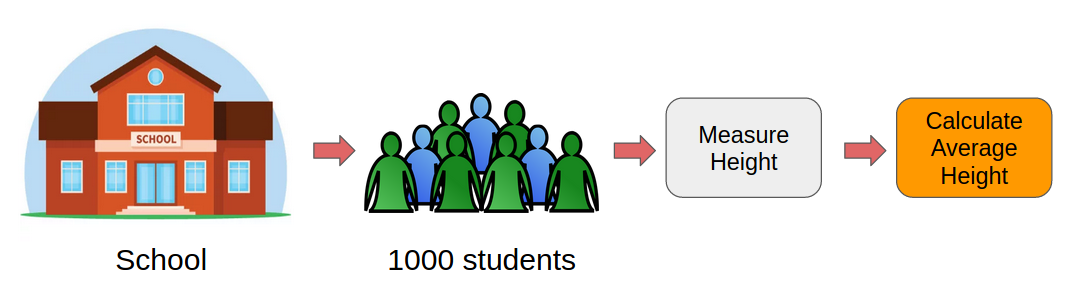However, this would be a tedious task. Just think about it, we would have to individually measure the heights of 1,000 students and then compute the mean height. It will take days! We need a smarter approach here.

This is where Bootstrap Sampling comes into play.

Instead of measuring the heights of all the students, we can draw a random sample of 5 students and measure their heights. We would repeat this process 20 times and then average the collected height data of 100 students (5 x 20). This average height would be an estimate of the mean height of all the students of the school.

Pretty straightforward, right? This is the basic idea of Bootstrap Sampling.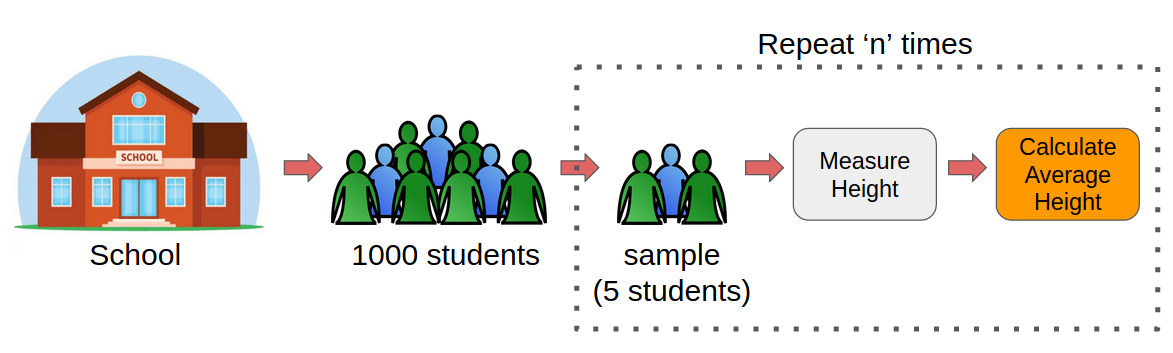Hence, when we have to estimate a parameter of a large population, we can take the help of Bootstrap Sampling.

## Bootstrap Sampling in Machine Learning

Bootstrap sampling is used in a machine learning ensemble algorithm called bootstrap aggregating (also called bagging). It helps in avoiding overfitting and improves the stability of machine learning algorithms.

In bagging, a certain number of equally sized subsets of a dataset are extracted with replacement. Then, a machine learning algorithm is applied to each of these subsets and the outputs are ensembled as I have illustrated below: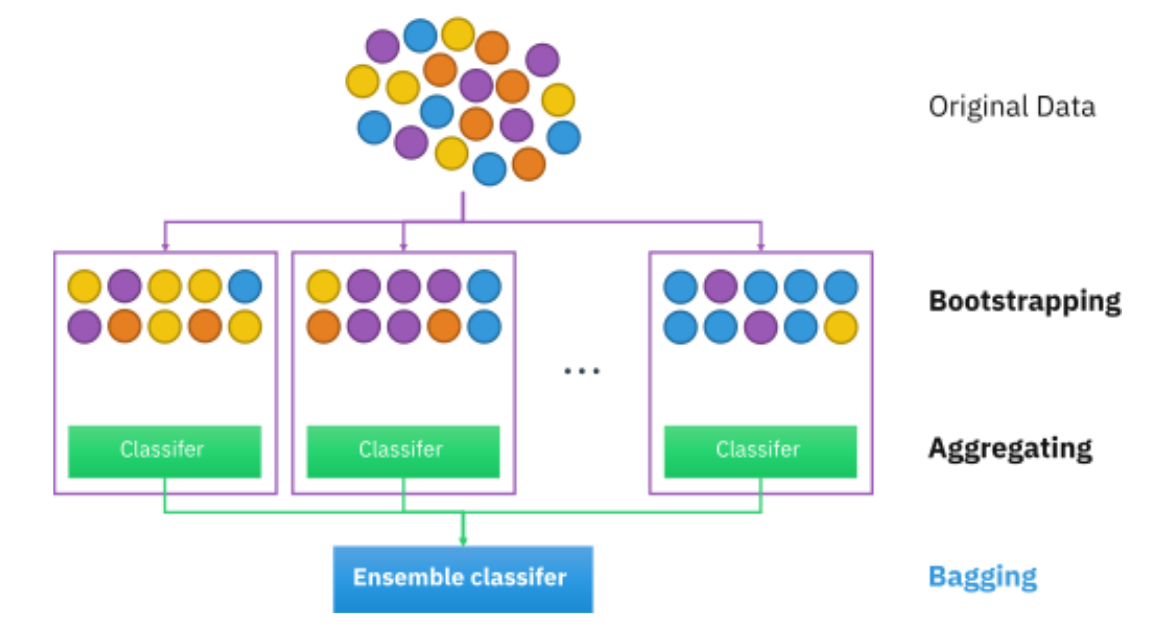## Implement Bootstrap Sampling in Python

Time to put our learning to the test and implement the concept of Bootstrap Sampling in Python.

In this section, we will try to estimate the population mean with the help of bootstrap sampling. Let’s import the required libraries:

Next, we will create a Gaussian distribution (population) of 10,000 elements with the population mean being 500:

Output: 500.00889503613934

Now, we will draw 40 samples of size 5 from the distribution (population) and compute the mean for every sample:

Let’s check the average of the mean values of all the 40 samples:

`np.mean(sample_mean)`

Output: 500.024133172629

It turns out to be pretty close to the population mean! This is why Bootstrap Sampling is such a useful technique in statistics and machine learning.

## Summarizing what we’ve Learned

In this article, we learned about the utility of Bootstrap Sampling in statistics and machine learning. We also implemented it in Python and verified it’s effectiveness.

Here are a few key benefits of bootstrapping:

• The estimated parameter by bootstrap sampling is comparable to the actual population parameter
• Since we only need a few samples for bootstrapping, the computation requirement is very less
• In Random Forest, the bootstrap sample size of even 20% gives a pretty good performance as shown below: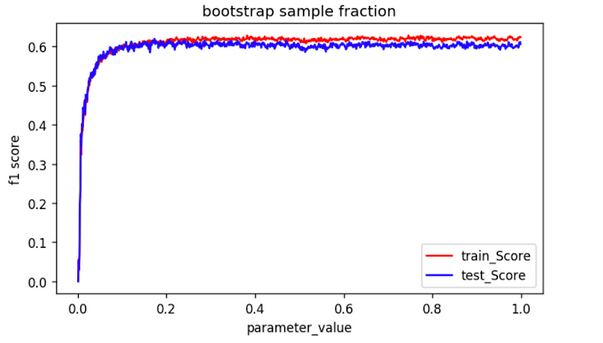The model performance reaches maximum when the data provided is less than 0.2 fraction of the original dataset.

You can also read this article on Analytics Vidhya's Android APP